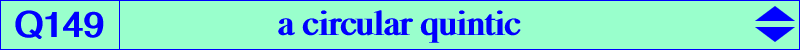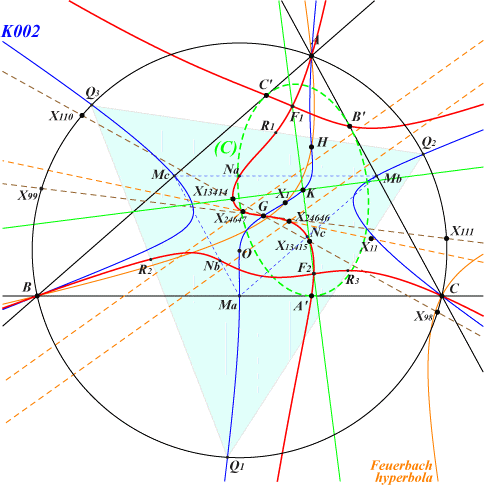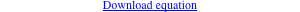too complicated to be written here. Click on the link to download a text file.X(2), X(13414), X(13415), X(24646), X(24647) vertices of the orthic triangle vertices of the orthic triangle of the medial triangle vertices of the orthic triangle of the Thomson triangle infinite points of K002 foci of the orthic inconic (C)Let S be a variable point on the circumcircle (O). Let (H) be the rectangular circum-hyperbola passing through S and (D) the diagonal hyperbola passing through the in/excenters of ABC and homothetic to (H). Denote by Oh and Od the respective centers of (H) and (D). Od lies on (O) and Oh is the complement of Od hence on the nine points circle. Let (Lo) be the line passing through these centers and X(2). (H) and (D) meet at two (real or not) finite points H1, H2 lying on the polar line (L) of X(2) in (D). (L) passes through X(6) and meets the Thomson cubic K002 at H1, H2 which are therefore X(2)-Ceva conjugates. These points also lie on the bicevian conic (B) = C(X2, T) where T is the trilinear pole of (L). The circle with diameter H1, H2 meets (Lo) at M1, M2 and when S describes (O), the locus of these points is Q149. Remarks : • the four foci of the orthic inconic (C) are nodes on the curve. • the tangents at A, B, C meet at X(2) which is a point of inflexion with inflexional tangent passing through X(99), X(111), X(115), etc. • X(2) is also the singular focus of Q149. • when (H) is the Jerabek hyperbola, (Lo) is the line X(2)X(98) and M1, M2 are X(13414), X(13415). • when (H) is the Feuerbach hyperbola, (Lo) is the line X(2)X(11) and M1, M2 are X(24646), X(24647). • when (H) is the Kiepert hyperbola, (Lo) is the line X(2)X(99)X(111) and M1, M2 coincide in X(2). *** A connection with Table 62 For a given point S as above, consider the pencil of rectangular hyperbolas generated by (H) and (D). The pole M* of M in this pencil may be seen as the isogonal conjugate of M in a non proper triangle (T) whose vertices are M1, M2 and the infinite point M0 of (L). H1, H2 and the infinite points of the parallels to (L) at M1, M2 may be seen as the in/excenters of (T). Now, for any P not lying on the sidelines of (T), the locus of M such that P, M, M* are collinear is an isogonal pK with respect to (T). This pK must pass through P, P*, the singular points M0, M1, M2 and the fixed points (the in/excenters above) of the isoconjugation, also the vertices of the cevian triangle of P in (T) which are M0* = M1M2 /\ PM0, M1* = PM1 /\ M0M2, M2* = PM2 /\ M0M1. Example 1 : S = X(74), (H) and (D) are the Jerabek and Stammler hyperbolas, (Lo) is the Brocard axis, (B) is the bicevian conic C(X2, X110) passing through X(i) for i in {3, 5, 6, 113, 141, 206, 942, 960, 1147, 1209, 1493, 1511, 2574, 2575, 2883, etc}. When P = X(51), the pK is K907. Example 2 : S = X(104), see K1083.